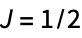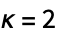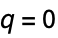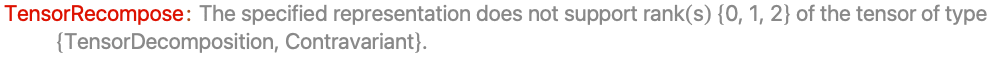AtomicDensityMatrix`
AtomicDensityMatrix`

# Recomposition

Recomposition[decomp]

returns the reducible operator corresponding to tensor decomposition decomp represented on a space with smallest angular momentum sufficient to support all moments.

Recomposition[j,decomp]

returns the reducible operator on a space with angular momentum j corresponding to tensor decomposition decomp.

Recomposition[sys,mat]

returns the operator for atomic system sys corresponding to the matrix of tensor decompositions mat.

Recomposition[j,tens]

returns the operator that has the spherical tensor tens as the only nonzero component of its tensor decomposition.

# Details and Options

• A tensor decomposition is a list of spherical tensors of increasing rank.

# Examples

open allclose all

## Basic Examples(2)

A tensor decomposition:

Construct the reducible operator in arepresentation:

An operator on aspace that contains only the,polarization moment in its decomposition:

## Properties & Relations(3)

Recomposition undoes Decomposition:

Decomposition undoes Recomposition:

The Recomposition of the Hermitian conjugate is the Hermitian conjugate of the Recomposition:

## Possible Issues(1)

Ifis specified, it must be at least twice the highest-rank polarization moment to be recomposed: